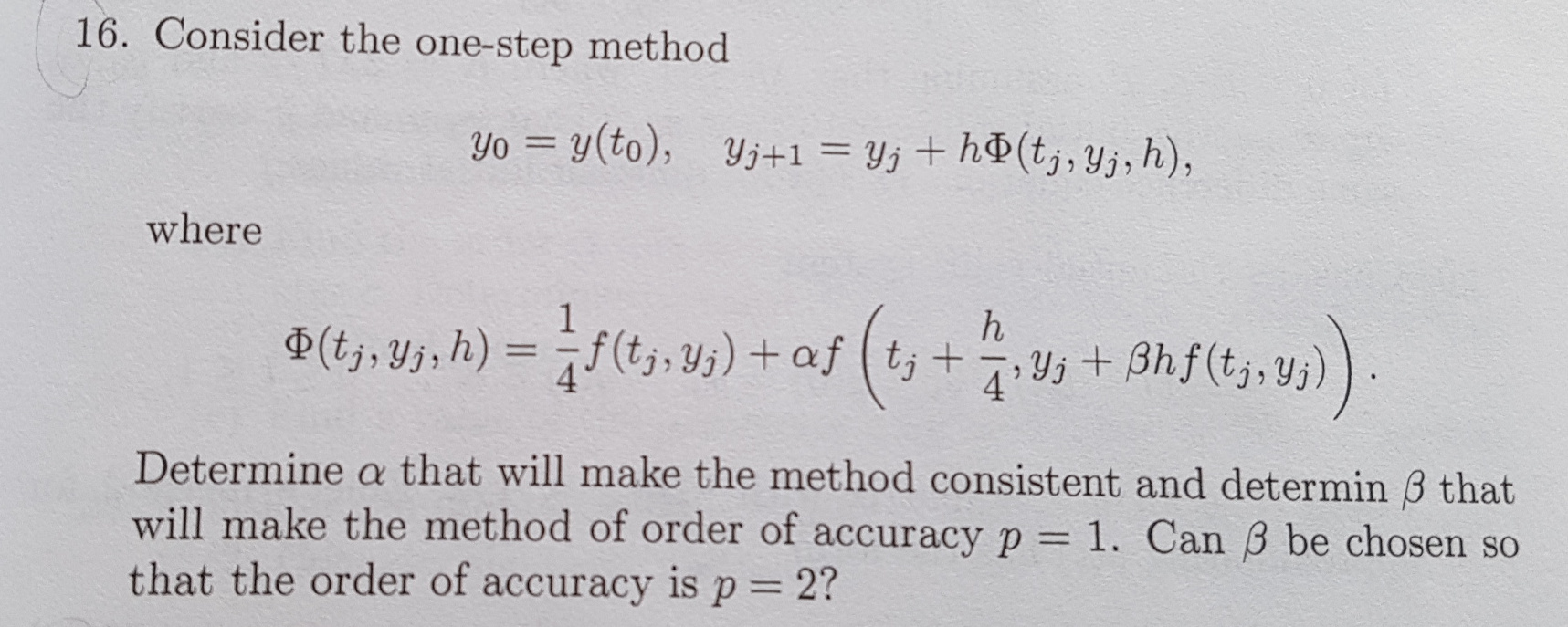# 1.¶

## Ackleh et al. Exercise 7.10.12¶

Find the equations that must be satisfied by the coefficients of a 3rd-order 3-stage Runge-Kutta method. Find a particular set of coefficients that satisfies these equations (ideally one that is not in Wikipedia!).

### Hints¶

Get a large quantity of paper, pencil and eraser, and a cup of strong coffee for this one: it is a heavy calculation.

Do it as follows. Consider the generic $i^{th}$ component of the solution of a 2D autonomous system $y^\prime = f(y)$. Making it autonomous reduces the complexity of the expressions, and doing it for 2D only keeps the expressions manageable but it will still be clear how they generalize to $n$ dimensions.

The idea is to write Taylor expansions for the values of $f_i$ in the Runge-Kutta formula, and match these with the Taylor expansion of $y_i(t_k+h)$. Your terms of your expansions will all be products of the RK coefficients, derivatives of the components $f_j$ of $f$, and the components $f_j$ themselves - all evaluated at the same point, so you don't need to waste time and ink writing "$(y^{\{k\}})$" to say they are evaluated at $y^{\{k\}}$, the current latest point of our numerical solution. I recommend using the compact notation $\partial_p f_q$ to denote the derivative of the $q^{th}$ component of $f(y)$ with respect to the $p^{th}$ component of y (at the point $y^{\{k\}}$).

The ingredients you will need are: (i) the differential equation itself, (ii) the chain rule of partial differentiation, and (iii) the multivariable Taylor expansion as given in Wikipedia, written out explicitly for 2 variables. You will not need to go beyond 2nd partials of the components of $f$.

Try not to waste time figuring out terms that will contribute at order higher than $h^3$.

# 2.¶

## Similar to Ackleh et al. Exercise 7.10.13¶

(a) Calculate the real part of the region of absolute stability of the a 3rd-order 3-stage Runge-Kutta method of your choice.

(b) Also make a picture of the stability region in the complex plane by modify the code I provided Day 6.

# 3.¶

## Ackleh et al. Exercise 7.10.16¶

In :
from IPython.display import Image
Image('Ackleh_ex_7.10.16.jpg',width=600)

Out:In [ ]: